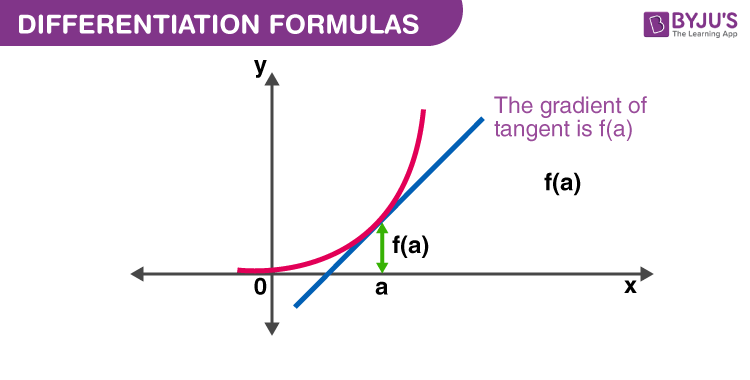# Differentiation Formulas

A Differentiation formulas list has been provided here for students so that they can refer to these to solve problems based on differential equations. This is one of the most important topics in higher class Mathematics. The general representation of the derivative is d/dx.

This formula list includes derivative for constant, trigonometric functions, polynomials, hyperbolic, logarithmic functions, exponential, inverse trigonometric functions etc. Based on these, there are a number of examples and problems present in the syllabus of Class 11 and 12, for which students can easily write answers.## Differentiation Formulas List

In all the formulas below, f’ means $\frac{d(f(x))}{dx} = f'(x)$ and g’ means $\frac{d(g(x))}{dx}$ = $g'(x)$ . Both f and g are the functions of x and differentiated with respect to x. We can also represent dy/dx = Dx y. Some of the general differentiation formulas are;

1. Power Rule: (d/dx) (xn )nxn-1
2. Derivative of a constant, a:  (d/dx) (a) = 0
3. Derivative of a constant multiplied with function f: (d/dx) (a. f)af’
4.  Sum Rule: (d/dx) (f ± g) = f’ ± g’
5. Product Rule: (d/dx) (fg)= fg’ + gf’
6. Quotient Rule:$\frac{d}{dx}(\frac{f}{g})$ = $\frac{gf’ – fg’}{g^2}$

### Differentiation Formulas for Trigonometric Functions

Trigonometry is the concept of relation between angles and sides of triangles. Here, we have 6 main ratios, such as, sine, cosine, tangent, cotangent, secant and cosecant. You must have learned about basic trigonometric formulas based on these ratios. Now let us see, the formulas for derivative of trigonometric functions.

1. $\frac{d}{dx} (sin~ x)= cos\ x$
2. $\frac{d}{dx} (cos~ x)= – sin\ x$
3. $\frac{d}{dx} (tan ~x)= sec^{2} x$
4. $\frac{d}{dx} (cot~ x = -cosec^{2} x$
5. $\frac{d}{dx} (sec~ x) = sec\ x\ tan\ x$
6. $\frac{d}{dx} (cosec ~x)= -cosec\ x\ cot\ x$
7. $\frac{d}{dx} (sinh~ x)= cosh\ x$
8. $\frac{d}{dx} (cosh~ x) = sinh\ x$
9. $\frac{d}{dx} (tanh ~x)= sech^{2} x$
10. $\frac{d}{dx} (coth~ x)=-cosech^{2} x$
11. $\frac{d}{dx} (sech~ x)= -sech\ x\ tanh\ x$
12. $\frac{d}{dx} (cosech~ x ) = -cosech\ x\ coth\ x$

### Differentiation Formulas for Inverse Trigonometric Functions

Inverse trigonometry functions are the inverse of trigonemetric ratios. Let us see the formulas for derivative of inverse trigonometric functions.

1. $\frac{d}{dx}(sin^{-1}~ x)$ = $\frac{1}{\sqrt{1 – x^2}}$
2. $\frac{d}{dx}(cos^{-1}~ x)$ = $-\frac{1}{\sqrt{1 – x^2}}$
3. $\frac{d}{dx}(tan^{-1}~ x)$ = $\frac{1}{1 + x^2}$
4. $\frac{d}{dx}(cot^{-1}~ x)$ = $-\frac{1}{1 + x^2}$
5. $\frac{d}{dx}(sec^{-1} ~x)$= $\frac{1}{|x|\sqrt{x^2 – 1}}$
6. $\frac{d}{dx}(cosec^{-1}~x)$= $-\frac{1}{|x|\sqrt{x^2 – 1}}$

### Other Differentiation Formulas

1. $\frac{d}{dx}(a^{x}) = a^{x} ln a$
2. $\frac{d}{dx}(e^{x}) = e^{x}$
3. $\frac{d}{dx}(log_a~ x)$ = $\frac{1}{(ln~ a)x}$
4. $\frac{d}{dx}(ln~ x) = 1/x$
5. Chain Rule: $\frac{dy}{dx}$ = $\frac{dy}{du} × \frac{du}{dx}$ = $\frac{dy}{dv} × \frac{dv}{du} × \frac{du}{dx}$

Bookmark this page and visit whenever you need a sneak peek at differentiation formulas. Also, visit us to learn integration formulas with proofs.  Download the BYJU’S app to get interesting and personalised videos and have fun learning.

 Related Links Differentiation Differentiation Integration Differential Equation Differential Equations Applications

1. Bhumika

Best and thanks alot I can easily learn all the formulas

I have use this I feel so easy to learn thanks

3. Vinay

Tqsm for providing these formulas

4. Puneet

Thanks for telling us these formulas

5. daya

thank u so much for the formulasss, it will really help meeeeee